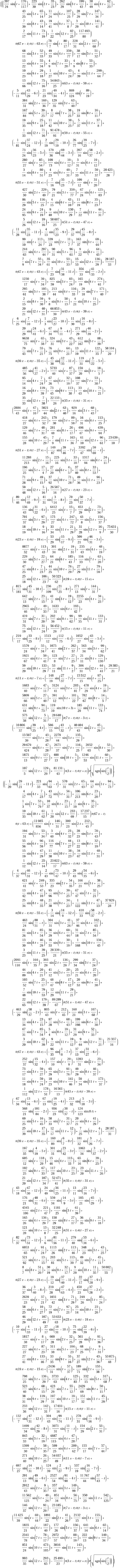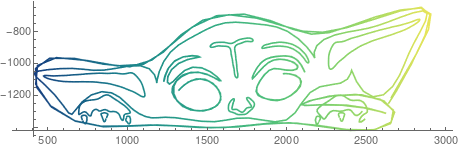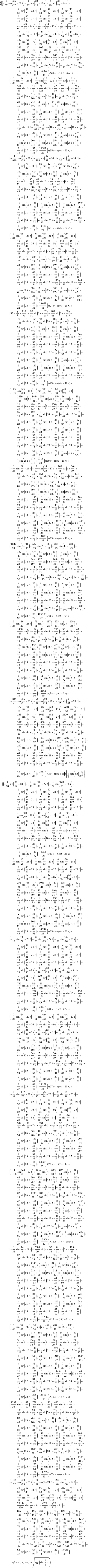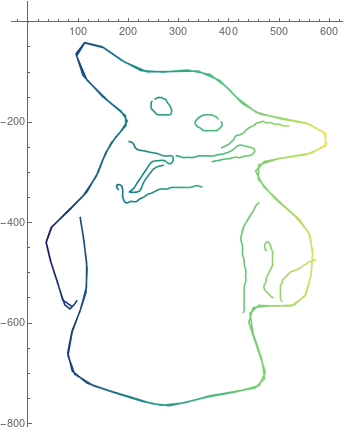# Baby Yoda’s Plot

Published:

After enjoying the incredible season 2 finale of The Mandalorian I was inspired to represent baby yoda in his truest form: mathematical.If you are a sane human and have no idea how these1 parametric equations2 are baby yoda, then here is their wonderful plot:And in the sake of the Christmas spirit here is another.which looks like:### Footnotes

1 I had quite a difficult time getting the equation to display directly in the browser and even $$\LaTeX$$ for that matter, hence, I put the ginormous image file in the middle of the page. So here are the equation’s .tex files for the first and second equations if for some odd reason you want them.

2 In both of the above set of parametric equations we have $$0\le t \le 36\pi$$ and $$\theta(\cdot)$$ is the Heaviside step function

Tags:

Categories: